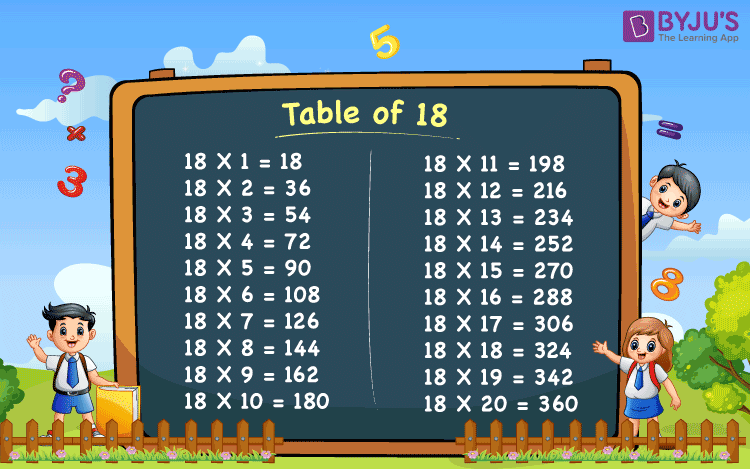# Table of 18

Table of 18 is considered one of the most complicated Maths tables below 20. 18 table represents the repeated addition of the number 18 with itself. The repeated addition of 18 to itself will depend on the number multiplied by it. For example, 18 multiplied by 3 is equal to 18 + 18 + 18 = 54. The 18 times table values are a bit difficult to remember and are hard to calculate. Here, the 18th multiplication table is given to help the students check the values quickly and memorize them.

## Table of 18 Chart

The table of 18 is given below, both in tabular form and in image form so that the students can download and use the table as a flashcard and memorize it more effectively. The 18 times table is extremely important for the students as it will help them to do mental calculations quickly and more efficiently.## 18 Times Table up to 20

Multiplication table of 18 up to 20 times is provided here. Students can memorise the table and practice multiplication problems based on them.

 18 × 1 = 18 18 × 2 = 36 18 × 3 = 54 18 × 4 = 72 18 × 5 = 90 18 × 6 = 108 18 × 7 = 126 18 × 8 = 144 18 × 9 = 162 18 × 10 = 180 18 × 11 = 198 18 × 12 = 216 18 × 13 = 234 18 × 14 = 252 18 × 15 = 270 18 × 16 = 288 18 × 17 = 306 18 × 18 = 324 18 × 19 = 342 18 × 20 = 360

## Tips to Remember 18 Table

18 is a two-digit number whose multiples are a little tricky to remember. Therefore, to memorise the table of 18, you can try these tips and learn quickly.

1. Double the table of 9 to get the table of 18.

 9  x  1  =  9 9  x  2 18 9  x  2  =  18 18  x  2 36 9  x  3  =  27 27  x  2 54 9  x  4  =  36 36  x  2 72 9  x  5  =  45 45  x  2 90 9  x  6  =  54 54  x  2 108 9  x  7  =  63 63  x  2 126 9  x  8  =  72 72  x  2 144 9  x  9  =  81 81  x  2 162 9  x  10  =  90 90  x  2 180

In the third column of the above table, we have got the 18 times table. Thus, if you remember table of 9, then you can easily remember the table of 18.

2. Subtract table of 2 from table of 20 to get table of 18.

 Table of 20 Table of 2 Subtraction Table of 18 20 2 20  –  2 18 40 4 40  –  4 36 60 6 60  –  6 54 80 8 80  –  8 72 100 10 100  –  10 90 120 12 120  –  12 108 140 14 140  –  14 126 160 16 160  –  16 144 180 18 180  –  18 162 200 20 200  –  20 180

## Solved Examples

Q.1: If Tina takes 18 minutes to complete 1 round of ground, how many minutes will she take to complete 4 rounds of the same ground?

Solution: Time taken by Tina to complete 1 round = 18 minutes

Time taken to complete 4 jobs = 18 x 4 = 72 minutes

Q.2: From the table of 18, find 18 times of 11.

Solution: 18 times of 11 = 18 x 11 = 198

Q.3: Find the value of a, if 18 x a = 126.

Solution: Given,

18 x a = 126

From the table of 18, we have;

18 x 7 = 126

Hence, the value of a is 7.

## Frequently Asked Questions on Table of 18

Q1

### What is a table of 18?

Table of 18 is the multiplication table that includes the multiples of 18.
Q2

### What is the value of 18 multiplied by 15?

18 multiplied by 15 is equal to 270.
Q3

### How to read 18 times table in English?

18 times table can be read as:
Eighteen ones are 18
Eighteen twos are 36
Eighteen threes are 54
Eighteen fours are 72
Eighteen fives are 90
and so on.
Q4

### What is Eighteen times of 10?

Eighteen times 10 is equal to 180. 18 x 10 = 180.

Keep visiting BYJU’S for more math’s tables and to learn several other important math’s topics in a more engaging and efficient way.

### More Multiplication Tables

Test your Knowledge on Table of 18